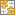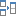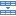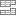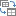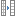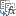# How to create a sequence of dates in Excel and auto fill date series

The tutorial shows how you can leverage the new SEQUENCE function to quickly generate a list of dates in Excel and use the AutoFill feature to fill a column with dates, workdays, months or years.

Until recently, there has been just one easy way to generate dates in Excel - the AutoFill feature. The introduction of the new dynamic array SEQUENCE function has made it possible to make a series of dates with a formula too. This tutorial takes an in-depth look at both methods so that you can choose the one that works best for you.

## How to fill date series in Excel

When you need to fill a column with dates in Excel, the fastest way is to use the AutoFill feature.

### Auto fill a date series in Excel

Filling a column or row with dates that increment by one day is very easy:

1. Type the initial date in the first cell.
2. Select the cell with the initial date and drag the fill handle (a small green square at the bottom-right corner) down or to the right.

Excel will immediately generate a series of dates in the same format as the first date that you typed manually.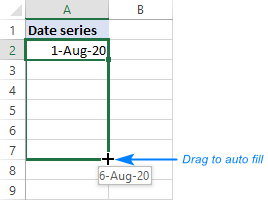### Fill a column with weekdays, months or years

To create a series of workdays, months or years, do one of the following:

• Fill a column with sequential dates as described above. After that, click the AutoFill Optionsbutton and pick the desired option, say Fill Months: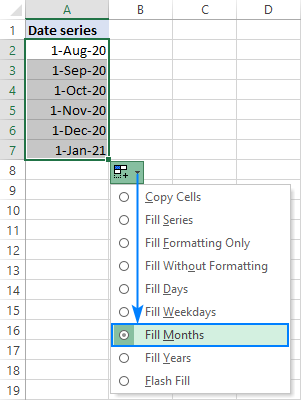• Or you can enter your first date, right-click the fill handle, hold and drag through as many cells as needed. When you release the mouse button, a context menu will pop-up letting you select the needed option, Fill Years in our case: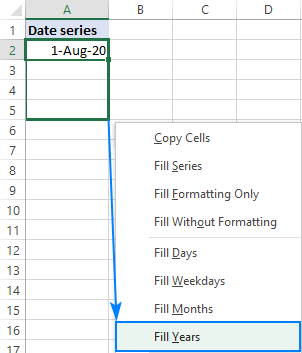### Fill a series of dates incrementing by N days

To auto generate a series of days, weekdays, months or years with a specific step, this is what you need to do:

1. Enter the initial date in the first cell.
2. Select that cell, right-click the fill handle, drag it through as many cells as needed, and then release.
3. In the pop-up menu, choose Series (the last item).
4. In the Series dialog box, select the Date unit of interest and set the Step value.
5. Click OK.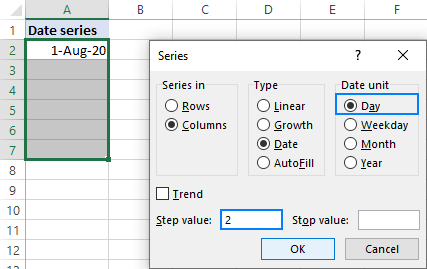## How to make a date sequence in Excel with a formula

In one of the previous tutorials, we looked at how to use the new dynamic array SEQUENCE function to generate a number sequence. Because internally in Excel dates are stored as serial numbers, the function can easily produce a date series too. All you have to do is to correctly configure the arguments as explained in the following examples.

Note. All the formulas discussed here only work in the latest versions of Excel 365 that support dynamic arrays. In pre-dynamic Excel 2019, Excel 2016 and Excel 2013, please use the AutoFill feature as shown in the first part of this tutorial.

### Create a series of dates in Excel

To generate a sequence of dates in Excel, set up the following arguments of the SEQUENCE function:

SEQUENCE(rows, [columns], [start], [step])
• Rows - the number of rows to fill with dates.
• Columns - the number of columns to fill with dates.
• Start - the starting date in the format that Excel can understand, like "8/1/2020" or "1-Aug-2020". To avoid mistakes, you can supply the date by using the DATE function such as DATE(2020, 8, 1).
• Step - the increment for each subsequent date in a sequence.

For example, to make a list of 10 dates starting with August 1, 2020 and increasing by 1 day, the formula is:

`=SEQUENCE(10, 1, "8/1/2020", 1)`

or

`=SEQUENCE(10, 1, DATE(2020, 8, 1), 1)`

Alternatively, you can input the number of dates (B1), start date (B2) and step (B3) in predefined cells and reference those cells in your formula. Since we are generating a list, the columns number (1) is hardcoded:

`=SEQUENCE(B1, 1, B2, B3)`

Type the below formula in the topmost cell (A6 in our case), press the Enter key, and the results will spill across the specified number of rows and columns automatically.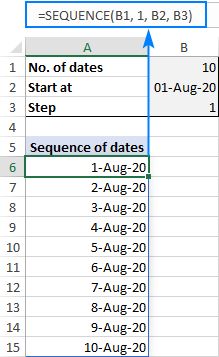Note. With the default General format, the results will appear as serial numbers. To have them displayed correctly, be sure to apply the Date format to all the cells in the spill range.

## Make a series of workdays in Excel

To get a series of working days only, wrap SEQUENCE in the WORKDAY or WORKDAY.INTL function this way:

WORKDAY(start_date -1, SEQUENCE(no_of_days))

As the WORKDAY function adds the number of days specified in the second argument to the start date, we subtract 1 from it to have the start date itself included in the results.

For instance, to generate a sequence of workdays starting on the date in B2, the formula is:

`=WORKDAY(B2-1, SEQUENCE(B1))`

Where B1 is the sequence size.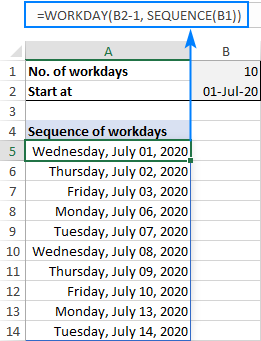Tips and notes:

• If a start date is Saturday or Sunday, the series will begin on the next working day.
• The Excel WORKDAY function assumes Saturday and Sunday to be weekends. To configure custom weekends and holidays, use the WORKDAY.INTL function instead.

## Generate a month sequence in Excel

To create a series of dates incremented by one month, you can use this generic formula:

DATE(year, SEQUENCE(12), day)

In this case, you put the target year in the 1st argument and day in the 3rd argument. For the 2nd argument, the SEQUENCE function returns sequential numbers from 1 to 12. Based on the above parameters, the DATE function produces a series of dates like shown in the left part of the screenshot below:

`=DATE(2020, SEQUENCE(12), 1)`

To display only the month names, set one of the below custom date formats for the spill range:

• mmm - short form like Jan, Feb, Mar, etc.
• mmmm - full form like January, February, March, etc.

As the result, only the month names will appear in cells, but the underlying values will still be full dates. In both series in the screenshot below, please notice the default right alignment typical for numbers and dates in Excel: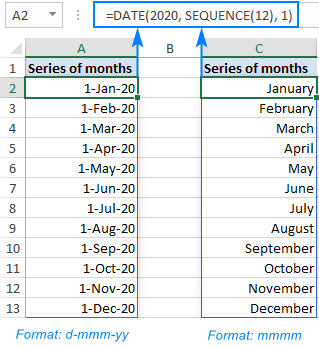To generate a date sequence that increments by one month and starts with a specific date, use the SEQUENCE function together with EDATE:

EDATE(start_date, SEQUENCE(12, 1, 0))

The EDATE function returns a date that is the specified number of months before or after the start date. And the SEQUENCE function produces an array of 12 numbers (or as many as you specify) to force EDATE to move forward in one-month increments. Please notice that the start argument is set to 0, so that the start date gets included in the results.

With the start date in B1, the formula takes this shape:

`=EDATE(B1, SEQUENCE(12, 1, 0))`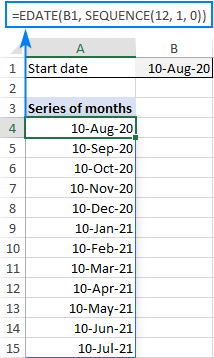Note. After completing a formula, please remember to apply an appropriate date format to the results for them to display correctly.

## Create a year sequence in Excel

To make a series of dates incremented by year, use this generic formula:

DATE(SEQUENCE(n, 1, YEAR(start_date)), MONTH(start_date), DAY(start_date))

Where n is the number of dates you want to generate.

In this case, the DATE(year, month, day) function constructs a date in this way:

• Year is returned by the SEQUENCE function that is configured to generate an n rows by 1 column array of numbers, starting at the year value from start_date.
• Month and day values are pulled directly from the start date.

For example, if you input the start date in B1, the following formula will output a series of 10 dates in one-year increments:

`=DATE(SEQUENCE(10, 1, YEAR(B1)), MONTH(B1), DAY(B1))`

After being formatted as dates, the results will look as follows: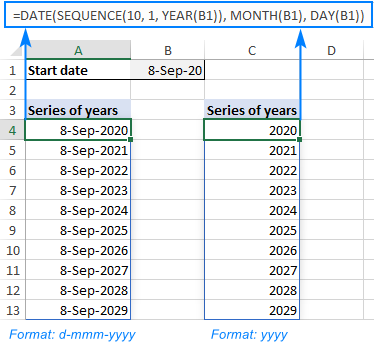## Generate a times sequence in Excel

Because times are stored in Excel as decimals numbers representing a fraction of the day, the SEQUENCE function can work with times directly.

Assuming the start time is in B1, you can use one of the following formulas to produce a series of 10 times. The difference is only in the step argument. As there are 24 hours in a day, use 1/24 to increment by an hour, 1/48 to increment by 30 minutes, and so on.

30 minutes apart:

`=SEQUENCE(10, 1, B1, 1/48)`

1 hour apart:

`=SEQUENCE(10, 1, B1, 1/24)`

2 hours apart:

`=SEQUENCE(10, 1, B1, 1/12)`

The screenshot below shows the results: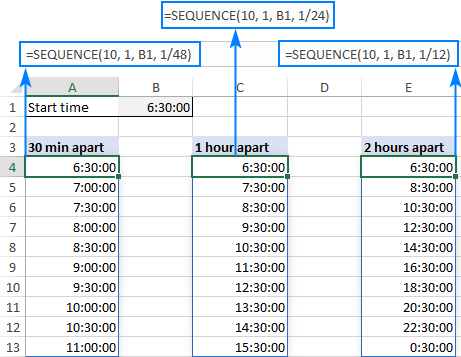If you do not want to bother calculating the step manually, you can define it by using the TIME function:

SEQUENCE(rows, columns, start, TIME(hour, minute, second))

For this example, we'll input all the variables in separate cells like shown in the screenshot below. And then, you can use the below formula to generate a time series with any increment step size you specify in cells E2 (hours), E3 (minutes) and E4 (seconds):

`=SEQUENCE(B2, B3, B4, TIME(E2, E3, E4))`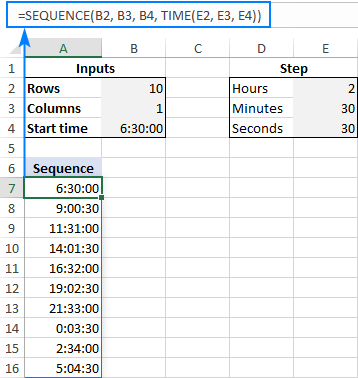## How to create a monthly calendar in Excel

In this final example, we'll be using the SEQUENCE function together with DATEVALUE and WEEKDAY to create a monthly calendar that will update automatically based on the year and month that you specify.

The formula in A5 is as follows:

`=SEQUENCE(6, 7, DATEVALUE("1/"&B2&"/"&B1) - WEEKDAY(DATEVALUE("1/"&B2&"/"&B1)) + 1, 1)`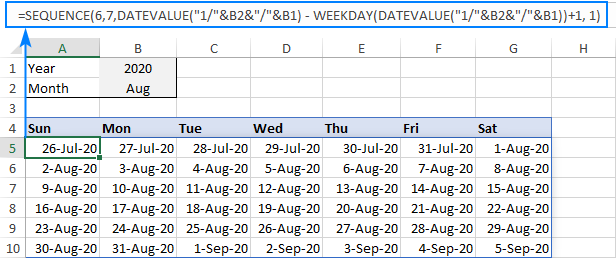How this formula works:

You use the SEQUENCE function to generate a 6 rows (the max possible number of weeks in a month) by 7 columns (the number of days in a week) array of dates incremented by 1 day. Hence, the rows, columns and step arguments raise no questions.

The trickiest part in the start argument. We cannot start our calendar with the 1st day of the target month because we do not know which day of the week it is. So, we use the following formula to find the first Sunday before the 1st day of the specified month and year:

`DATEVALUE("1/"&B2&"/"&B1) - WEEKDAY(DATEVALUE("1/"&B2&"/"&B1)) + 1`

The first DATEVALUE function returns a serial number that, in the internal Excel system, represents the 1st day of the month in B2 and the year in B1. In our case, it's 44044 corresponding to August 1, 2020. At this point, we have:

`44044 - WEEKDAY(DATEVALUE("1/"&B2&"/"&B1)) + 1`

The WEEKDAY function returns the day of the week corresponding to the 1st day of the target month as a number from 1 (Sunday) to 7 (Saturday). In our case, it's 7 because August 1, 2020 is Saturday. And our formula reduces to:

`44044 - 7 + 1`

44044 - 7 is 4403, which corresponds to Saturday, July 25, 2020. As we need Sunday, we add the +1 correction.

This way, we get a simple formula that outputs an array of serial numbers beginning with 4404:

`=SEQUENCE(6, 7, 4404, 1)`

Format the results as dates, and you'll get a calendar shown in the screenshot above. For example, you can use one of the following date formats:

• d-mmm-yy to display dates like 1-Aug-20
• mmm d to display month and day like Aug 20
• d to display only the day

Wait, but we aim to create a monthly calendar. Why do some dates of the previous and next month show up? To hide away those irrelevant dates, set up a conditional formatting rule with the below formula and apply the white font color:

`=MONTH(A5)<>MONTH(DATEVALUE(\$B\$2 & "1"))`

Where A5 is the leftmost cell of your calendar and B2 is the target month.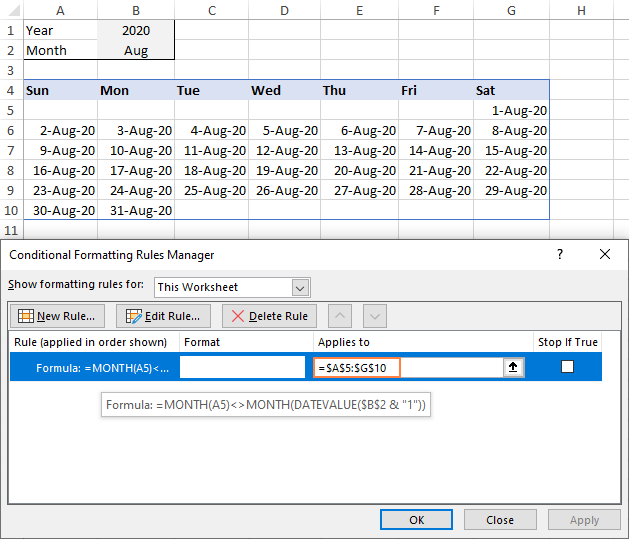For the detailed steps, please see How to create a formula-based conditional formatting rule in Excel.

That's how you can generate a sequence of dates in Excel. I thank you for reading and hope to see you on our blog next week!

Date sequence in Excel - formula examples (.xlsx file)

### One response to "How to create a sequence of dates in Excel and auto fill date series"

1.Ferris says:

This is so helpful, thank you!

Ultimate Suite for Microsoft Excel